# (t), For this question, this system has two inputs. The first input is the velocity of The following figure depicts...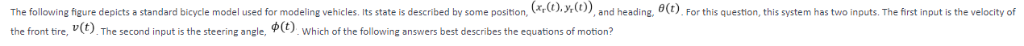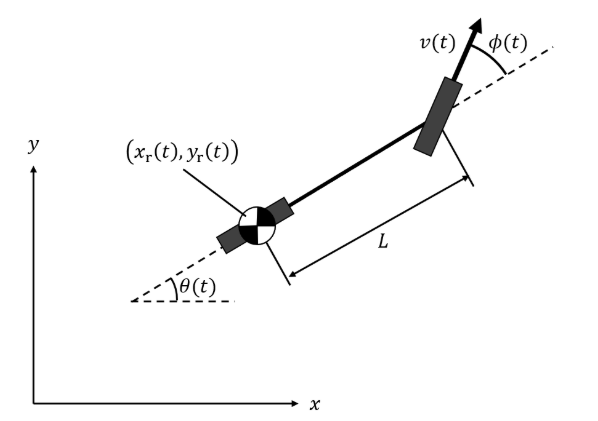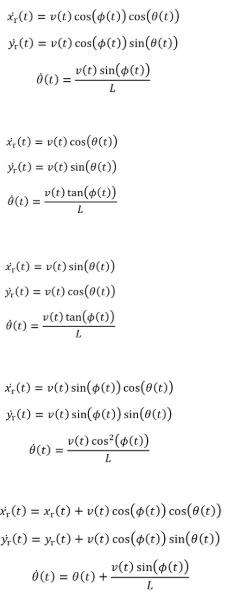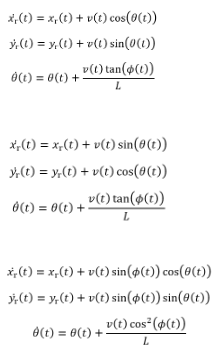(t), For this question, this system has two inputs. The first input is the velocity of The following figure depicts a standard bicycle model used for modeling vehicles. Its state is described by some position, r ф(t) the front tire, v(t). The second input is the steer which of the following answers best describes the equations of motion? the
(xr(t), yr(t))
4(1)-r(t) cos(φ(t)) cos(0(t)) (t)(t) cos(p())sin((t)) 0(1)-v(t)sin(φ(t)) ) (t)cos(o(t) A(t)-v(t) sin(θ (t)) 00-v(t) tan(φ(t)) A(t) 4(t)-v(t) sin(θ(t)) ))cos(o(o) v(t) tan(p(t)) ,(t)-v(t) sin(φ(t)) cos(θ(t)) It(t)-v(t)sin(d(t)) sin(θ(t)) θ(t)s u(t) cos*(φ(t)) +vt) cos(p(t)) cos(()) y(t)-y(t) + u(t) cos(φ(t)) sin(θ (t)) 0(t)s 0(t) + v(t)sin(φ(t))
))+()cos(oo)) ) )+ v)sin(o)) 0(t) + v(t)tan(φ(t)) 6(1) 4(t)-xr(t) + v(t) sin(θ(t)) )-)+v)cos((o) 0Otano )x+v()sin(p()) cos(e()) A(t)-y(t) + v(t) sin(d(t)) sin(θ(t)) 6(t) + v(t)cos2(φ(t)) θ(t)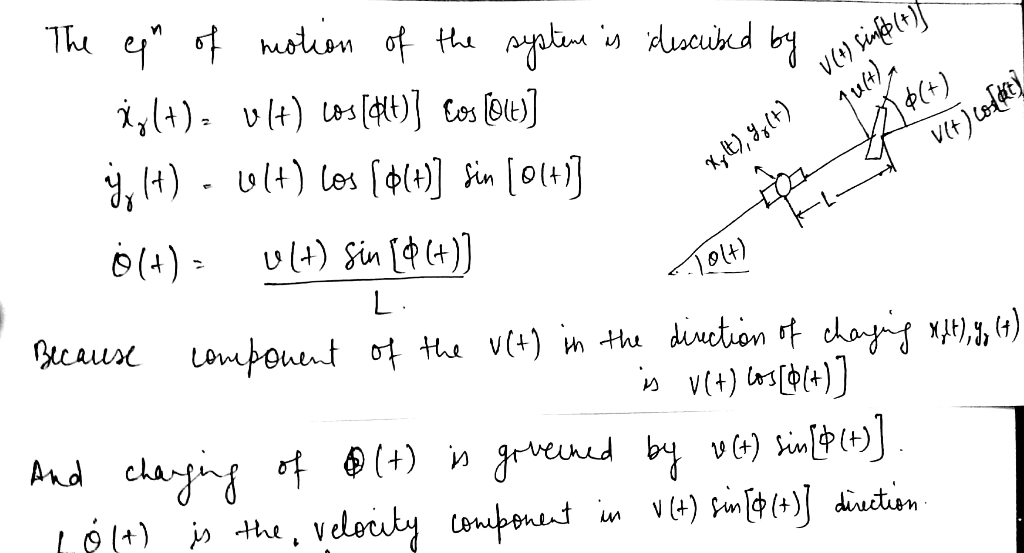##### Add Answer of: (t), For this question, this system has two inputs. The first input is the velocity of The following figure depicts...
Similar Homework Help Questions
• ### Consider the following surface parametrization. x-5 cos(8) sin(φ), y-3 sin(θ) sin(p), z-cos(p) Find an expression for a unit vector, n, normal to the surface at the image of a point (u, v) for θ in [...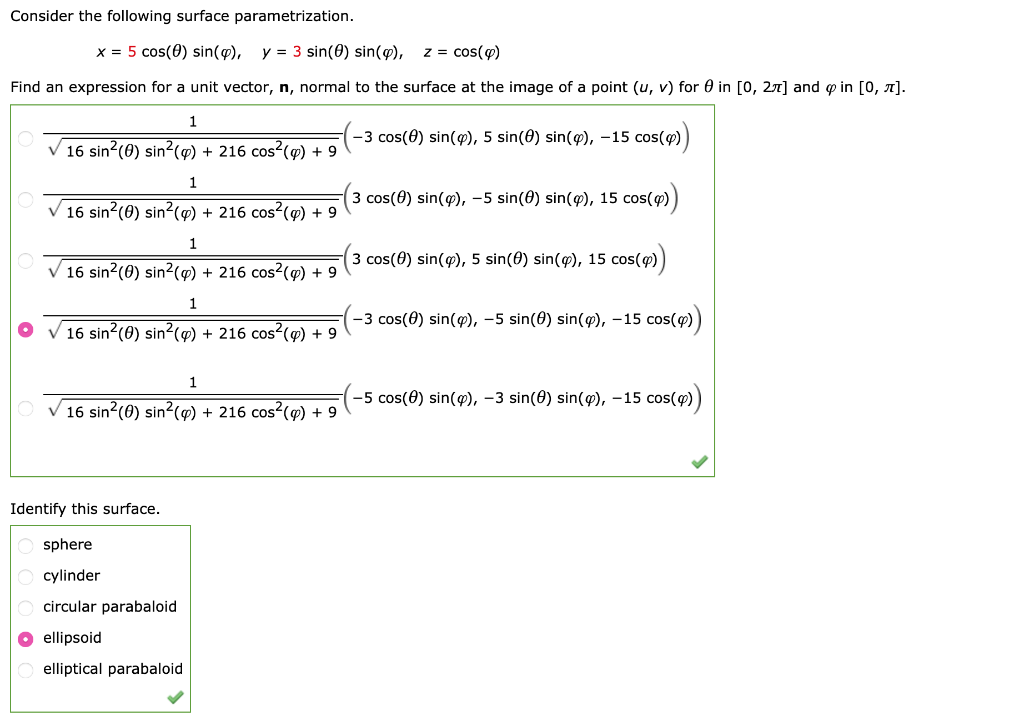Consider the following surface parametrization. x-5 cos(8) sin(φ), y-3 sin(θ) sin(p), z-cos(p) Find an expression for a unit vector, n, normal to the surface at the image of a point (u, v) for θ in [0, 2T] and φ in [0, π] -3 cos(θ) sin(φ), 5 sin(θ) sin(φ),-15 cos(q) 16 sin2(0) sin2(p)216 cos2(p)9 3 cos(9) sin(9),-5 sin(θ) sin(9), 15 cos(q) 16 sin2(0) sin2(p)216 cos2(p)9 v 16 sin2(0) [email protected] 216 [email protected](3 cos(θ) sin(φ), 5 sin(θ) sin(φ) , 15 cos(q) 216 cos(φ)...

• ### In the figure below, find each of the following. 5.00 3.00 4.00 (a) the side opposite...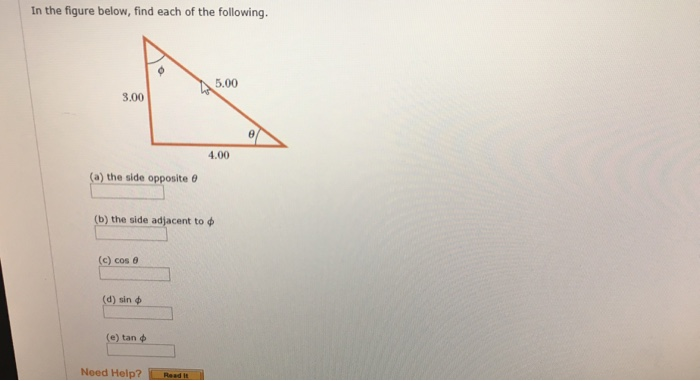In the figure below, find each of the following. 5.00 3.00 4.00 (a) the side opposite (b) the side-adjacent to ф (c) cos θ (d) sin ф e) tan For the triangle shown in the figure below what are each of the following? (Let y 60.0 m and r 65.0 m. Assume the triangle is a right trilangle.) a) the length of the unknown side x b) the tangent of o c)the sin of φ Need Help?

• ### please i need the question 15 for the detailed proof and explaination ! thanks ! 233 42 Isometries, Conformal Maps 14,...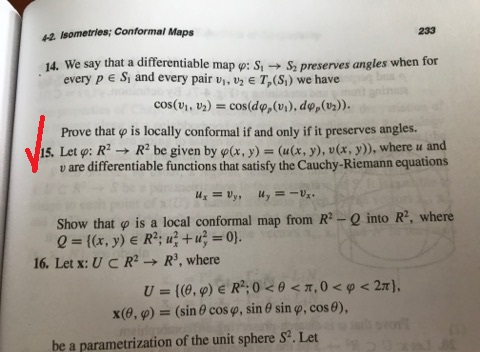please i need the question 15 for the detailed proof and explaination ! thanks ! 233 42 Isometries, Conformal Maps 14, we say that a differentiable map ф: S,--S2 preserves angles when for every p e Si and every pair vi, v2 E T (S,) we have cos(u, 2) cos(dp, (vi). do,()). Prove that pis locally conformal if and only if it preserves angles. 15. Letp: R2 R2 be given by ф(x, y)-(u (x, y), u(x, y), where u and...

• ### sin2 0+cos2 0=1 sin2 0=1-cos 20 cos2 0+cos 20 cos 20 cos2 0- sin2 sin 20...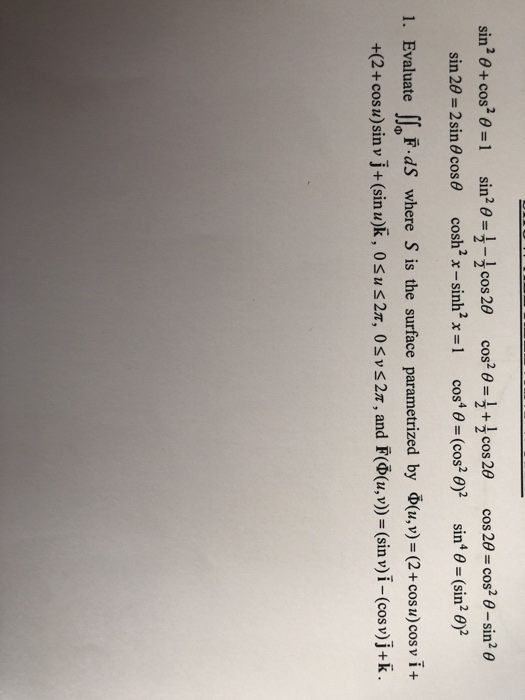sin2 0+cos2 0=1 sin2 0=1-cos 20 cos2 0+cos 20 cos 20 cos2 0- sin2 sin 20 2 sin 60 cos cosh2 x-sinh2 x =1 cos4 0 (cos2 0)2 sin 0= (sin2 0)2 1. Evaluate F dS where S is the surface parametrized by (u,v)= (2+ cos u) cos v i + +(2+ cosu)sin v j+(sin u)k, 0su 27, 0svs 27, and F((u,v))= (sin v)i -(cos v)j+k 2. Verify Green's Theorem for the function F(x, y) = (2y+3) i+(-5x)j where D...

• ### (a) (4 pts) LTI Systems. Consider two LTI subsystems that are connected in series, where system Tl has step response s(t)-1)-u(t-5) and system T2 has impulse response h2(t) e*u(t). Find the overall i...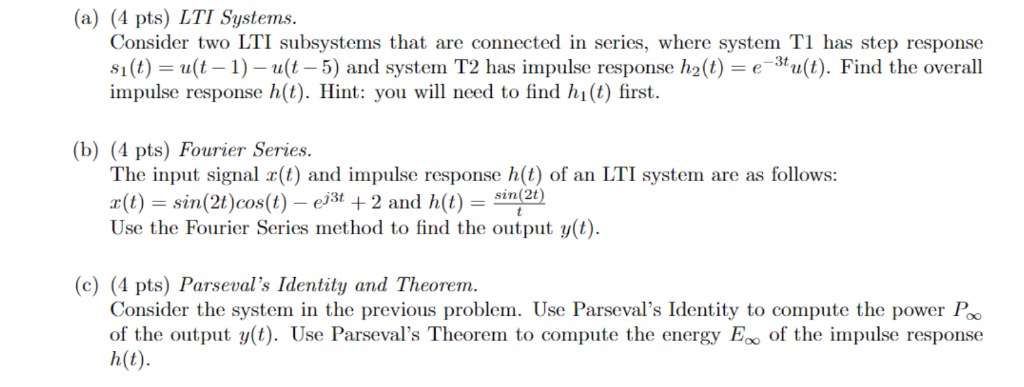(a) (4 pts) LTI Systems. Consider two LTI subsystems that are connected in series, where system Tl has step response s(t)-1)-u(t-5) and system T2 has impulse response h2(t) e*u(t). Find the overall impulse response h(t). Hint: you will need to find hi(t) first (b) (4 pts) Fourier Series. The input signal r(t) and impulse response h(t) of an LTI system are as follows: r(t) sin(2t)cos(t)e +2 and h(t) Use the Fourier Series method to find the output y(t) sin (2t)...

• ### questions are connected. please show steps to all Consider the system characterized by the characteristic polynomial...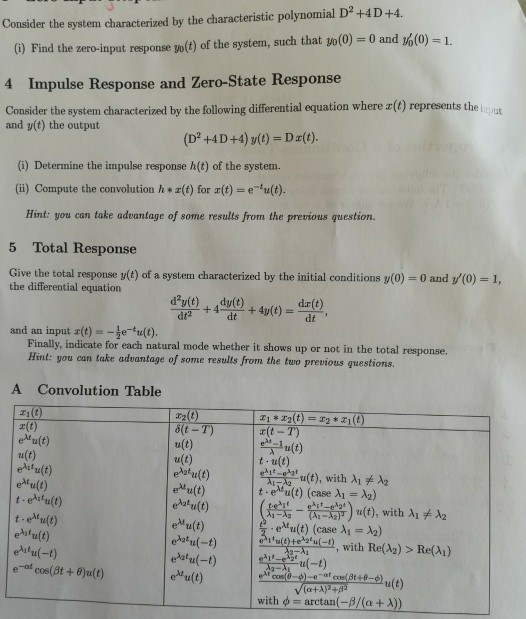questions are connected. please show steps to all Consider the system characterized by the characteristic polynomial D2+4D+4. (0) Find the zero-input response go(t) of the system, such that vol(o) 0 and o(0) -1. Impulse Response and Zero-State Response 4 Consider the system characterized by the following diferential equation where z() represents the iaut and y(t) the output (D2 +4D+4) y(t) Da(t) (i) Determine the impulse response h(t) of the system. (i) Compute the convolution h r(t) for r(t) eu(t). Hint:...

• ### D1 = 7 D2 = 4 Any assistance would be greatly appreciated Question 3 Left end (x 0) of a copper rod of length 100mm is kept at a constant temperature of Temp - 10+d2 degrees and the right end and...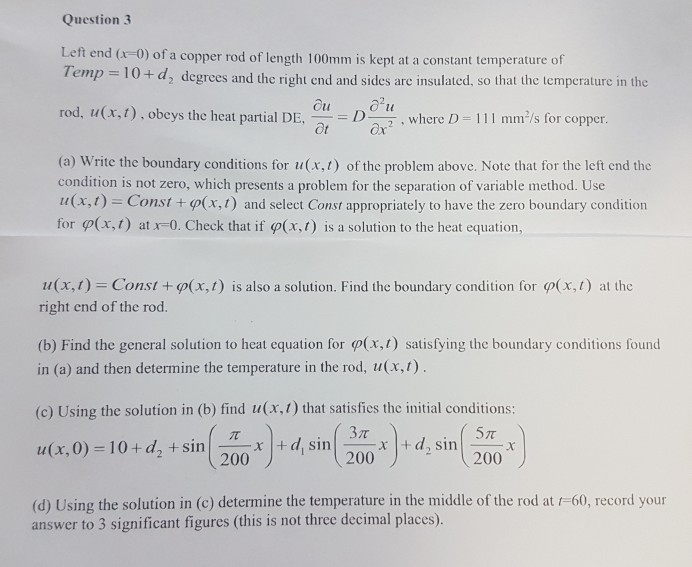D1 = 7 D2 = 4 Any assistance would be greatly appreciated Question 3 Left end (x 0) of a copper rod of length 100mm is kept at a constant temperature of Temp - 10+d2 degrees and the right end and sides are insulated, so that the temperature in the rod, u(x,t). obeys the heat partial DE, CD11 mms copper. where D-1 mm's for copper (a) Write the boundary conditions for u(x, 1) of the problem above. Note that for...

• ### 3. For following input/output system relationships, determine the impulse response h(t). Clearly show all the steps...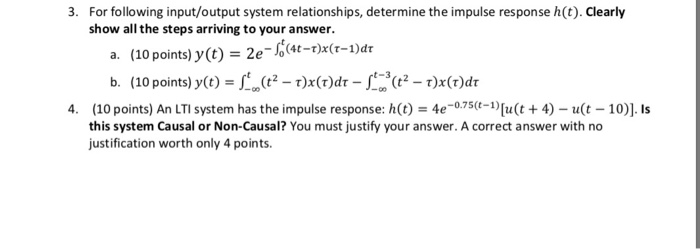3. For following input/output system relationships, determine the impulse response h(t). Clearly show all the steps arriving to your answer. p(-)x(1-)a L(2- r)x(1)dr-L*-1)x(1)dr (10 points) y(t) a. b. (10 points) y(t) -00 4. (10 points) An LTI system has the impulse response: h(t) = 4e-0.75(-1)[u(t + 4) - u(t - 10)]. this system Causal or Non-Causal? You must justify your answer. A correct answer with no justification worth only 4 points Is 3. For following input/output system relationships, determine the...

• ### The equations for the charge Q(t) and current i (t) in the following LRC circuit are Question 3 (...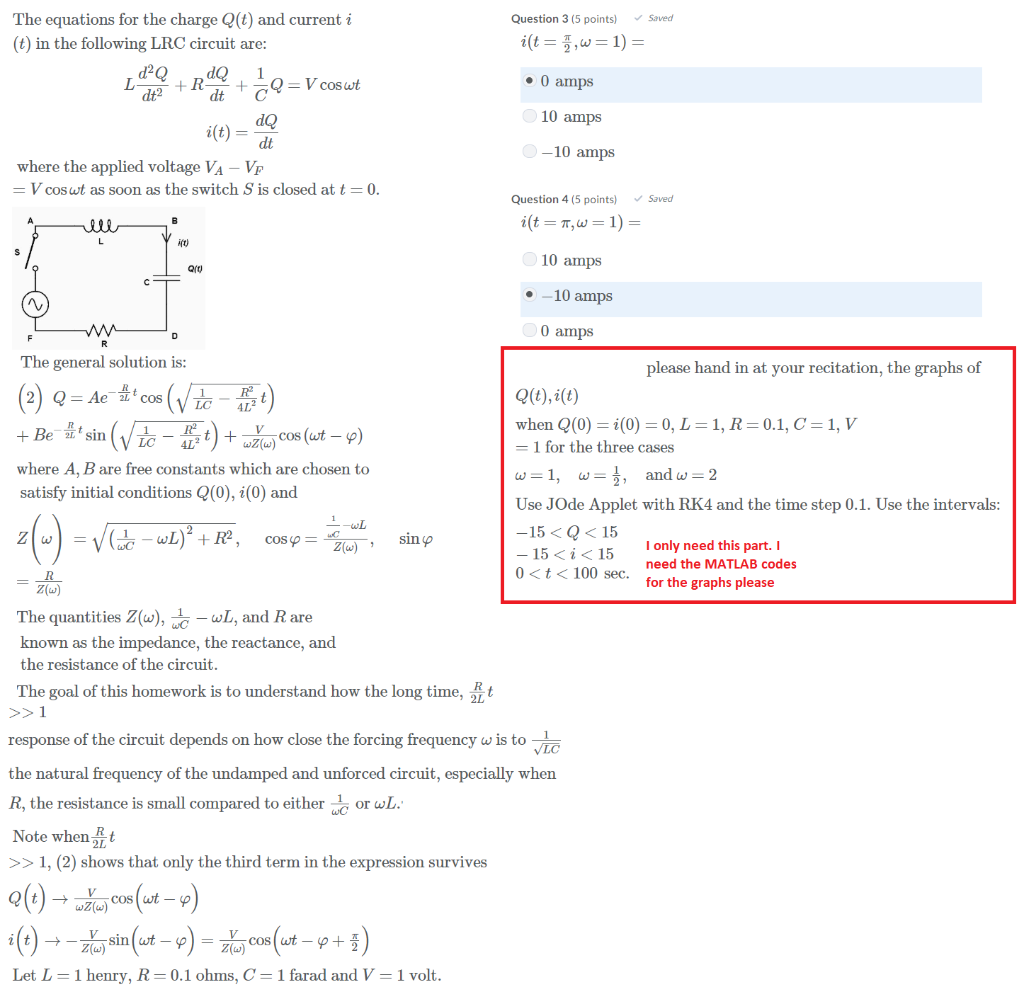3 The equations for the charge Q(t) and current i (t) in the following LRC circuit are Question 3 (5 points) Saved 0 amps dt C 10 amps i(t) dt 10 amps where the applied voltage VA -Vp V cos at as soon as the switch S is closed at t 0 Question 4 (5 points Saved ift) 10 amps -10 amps 0 amps Qrt The general solution is: please hand in at your recitation, the graphs of when Q(0)-i(0-0,...

• ### For Questions 3 and 4: the three spherical harmonics with 1-1 are given by ㄚㄧˇ条cos θ and Yitl...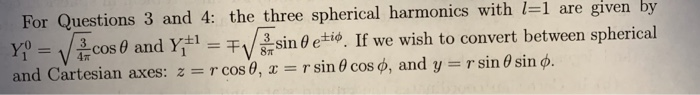For Questions 3 and 4: the three spherical harmonics with 1-1 are given by ㄚㄧˇ条cos θ and Yitlー干 in θ etio If we wish to convert between spherical and Cartesian axes: z r cos θ, x r sin θ coso, and y r sin θ sin φ. 4π Question 4: Consider the 3D simple harmonic oscillator potential: V(x,y,z)- 1mw2(x2 + y2 + z2). In class we solved this problem through Cartesian sep- aration of variables and found that the stationary...

Need Online Homework Help?